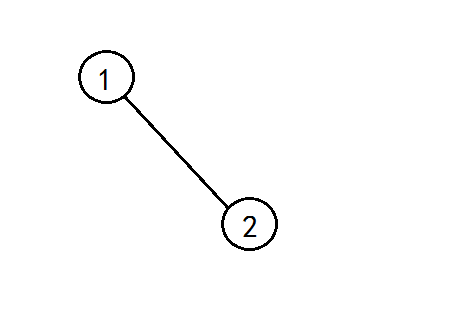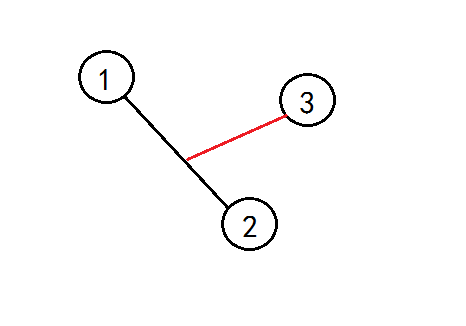# 题目分析

n=2 的时候，只有一条链。n=3 的时候，由于是一棵树，所以 3 肯定连在 1 到 2 的那条链上，那么红边长=(a(1,3)+a(2,3)-a(1,2))/2n=4 的时候，4 要么连在 1 到 2 的链上，要么连在 1 到 3 的链上（这样 2 到 3 的链已经包括在里面了），所以我们枚举并求最小新边权即可。

# 代码

#include<iostream>
#include<cstdio>
#include<algorithm>
#include<cstring>
#include<climits>
using namespace std;
int q=0;char ch=' ';
while(ch<'0'||ch>'9')ch=getchar();
while(ch>='0'&&ch<='9')q=q*10+ch-'0',ch=getchar();
return q;
}
int n,ans;
int a;
int main(){
int i,j;
while(1){
for(i=1;i<=n;++i)
for(j=i+1;j<=n;++j)
ans=a;
for(i=3;i<=n;++i){
int minn=INT_MAX;
for(j=2;j<i;++j)
minn=min(minn,(a[i][j]+a[i]-a[j])/2);
ans+=minn;
}
printf("%d\n",ans);
}
return 0;
}